Contrast Stretch

A contrast stretch is a linear piece-wise mapping of image gray values.  The slope of the function should be greater than 1 over the range of pixel values to be contrast stretched.  If an image has a lot of detail over a small range of pixel values, using a contrast stretch will spread those pixel values out while maintaining the shapes in the image.  One simple way to do this is to take the minimum value of an image and map it to zero.  Take the highest value and map it to 255 (the max gray value).  Since this is linear, all values in between are spread out accordingly.  Here are some examples.

Original                                                                        Contrast Stretch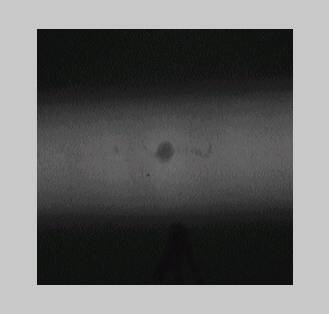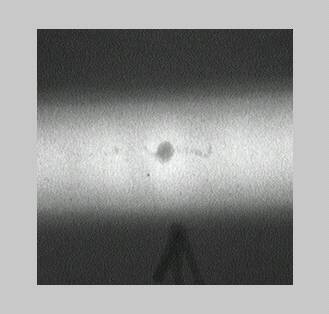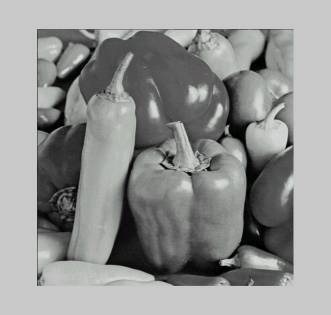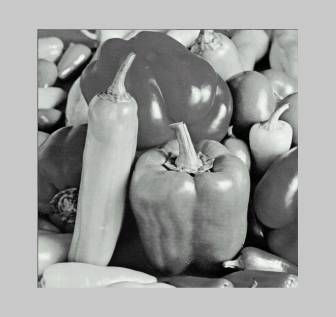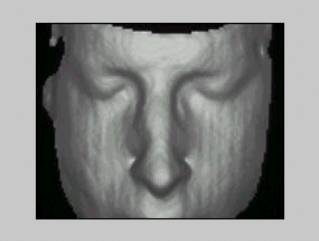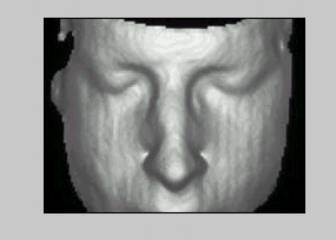As you can see, images with a smaller range of pixel values seem to change more.

There are two different ways to do a contrast stretch.  One way is to actually modify the image.  Another way is to change the lookup table.  I tried both and found (as expected) that modifying the lookup table is much faster.  Here are the results for top left image.

Direct Modification:

The median was .22 seconds (using matlab 5.3 tic and toc) and 458753 flops (using flop).

Table Modification:

The median was 0.0 seconds and 1787 flops.